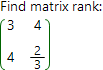# Matrix rank online calculatorMatrix rank is the number of lineary independent rows or columns. Therefore, to find matrix rank, one need simplify initial matrix by means of some elementary transformations which do not change the rank of initial matrix. After these transformations get done, one will receive new matrix with the same rank as initial. That's what our calculator does.

Matrix rank calculator
A: ×A =